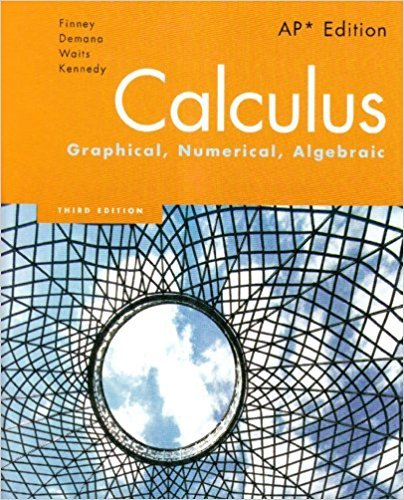×
×

# Solutions for Chapter 6: Calculus: Graphical, Numerical, Algebraic 3rd Edition## Full solutions for Calculus: Graphical, Numerical, Algebraic | 3rd Edition

ISBN: 9780132014083Solutions for Chapter 6

Solutions for Chapter 6
4 5 0 383 Reviews
23
0
##### ISBN: 9780132014083

Since 69 problems in chapter 6 have been answered, more than 4548 students have viewed full step-by-step solutions from this chapter. Calculus: Graphical, Numerical, Algebraic was written by and is associated to the ISBN: 9780132014083. Chapter 6 includes 69 full step-by-step solutions. This textbook survival guide was created for the textbook: Calculus: Graphical, Numerical, Algebraic, edition: 3. This expansive textbook survival guide covers the following chapters and their solutions.

Key Calculus Terms and definitions covered in this textbook
• Basic logistic function

The function ƒ(x) = 1 / 1 + e-x

• Binomial

A polynomial with exactly two terms

• Characteristic polynomial of a square matrix A

det(xIn - A), where A is an n x n matrix

• Finite series

Sum of a finite number of terms.

• Gaussian curve

See Normal curve.

• Identity matrix

A square matrix with 1’s in the main diagonal and 0’s elsewhere, p. 534.

• Inductive step

See Mathematical induction.

• Interval notation

Notation used to specify intervals, pp. 4, 5.

• Linear programming problem

A method of solving certain problems involving maximizing or minimizing a function of two variables (called an objective function) subject to restrictions (called constraints)

• Negative numbers

Real numbers shown to the left of the origin on a number line.

The measure of a central angle whose intercepted arc has a length equal to the circle’s radius.

The distance from a point on a circle (or a sphere) to the center of the circle (or the sphere).

• Scientific notation

A positive number written as c x 10m, where 1 ? c < 10 and m is an integer.

• Solve by elimination or substitution

Methods for solving systems of linear equations.

• Statistic

A number that measures a quantitative variable for a sample from a population.

• Sum of functions

(ƒ + g)(x) = ƒ(x) + g(x)

• Supply curve

p = ƒ(x), where x represents production and p represents price

• Trigonometric form of a complex number

r(cos ? + i sin ?)

• Variation

See Power function.

• y-intercept

A point that lies on both the graph and the y-axis.

×